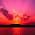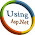# Introduction: Hello guys, in this article i will explain that how we can createVisualization: pie Chartusing asp.net. This is very helpful artucle for the asp.net deveploer to creating a mast graph in asp.net.

Implementation: Here i am taking the reference from the below link . http://code.google.com/apis/chart/interactive/docs/gallery/piechart.html
Google use javascript code for the implementation of this graph. I am using same javascript code but am giving also my asp.net experience with this code. And create the same graph in Asp.Net.

Code for .aspx page:
<title>Dynamic pie Chart</title>
<%--the below two javascripts  are needed to run this program you can

<body>
<form id="form1" runat="server">
<div>

<asp:Literal ID="lt" runat="server"></asp:Literal>
</div>
<div id="chart_div"></div>

</form>
</body>
Code for aspx.cs page:

using System;
using System.Collections;
using System.Configuration;
using System.Data;
using System.Linq;
using System.Web;
using System.Web.Security;
using System.Web.UI;
using System.Web.UI.HtmlControls;
using System.Web.UI.WebControls;
using System.Web.UI.WebControls.WebParts;
using System.Xml.Linq;
using System.Text;
using System.Data.SqlClient;

public partial class visulazitation_pie_chart : System.Web.UI.Page
{
// complete code of Visualization: Combo Chart
SqlConnection con = new SqlConnection();
// here am declairing the stringbuilder class
StringBuilder str = new StringBuilder();
protected void Page_Load(object sender, EventArgs e)
{
// here i am declare connection
con.ConnectionString = ConfigurationManager.ConnectionStrings["cn"].ConnectionString;
con.Open();
if (Page.IsPostBack == false)
{
// here i am calling function  chart_bind(); in the page load event of the page
chart_bind();
}

}
private void chart_bind()
{
// here i am using SqlDataAdapter for the sql server select query
// here am taking datatable
DataTable dt = new DataTable();
try
{
// here datatale dt is fill wit the adp
// this string m catching in the stringbuilder class
// in the str m writing same javascript code that is given by the google.

function drawChart() {
// but m changing  only below line
// my data that will come from the sql server
// here i am declairing the variable i in int32 for the looping statement
Int32 i;
// loop start from 0 and its end depend on the value inside dt.Rows.Count - 1
for (i = 0; i <= dt.Rows.Count - 1; i++)
{
// here i am fill the string builder with the value from the database
str.Append("['" + (dt.Rows[i]["student_name"].ToString()) + "'," + dt.Rows[i]["average_marks"].ToString() + "],");
}
// other all string is fill according to the javascript code
str.Append(" ]);");
str.Append(" var chart = new google.visualization.PieChart(document.getElementById('chart_div'));");
//  str.Append(" var chart = new google.visualization.BarChart(document.getElementById('chart_div'));");
str.Append("chart.draw(data, {width: 1200, height: 500,title: 'Students Record'});}");
str.Append("</script>");
// here am using literal conrol to display the complete graph
lt.Text = str.ToString().TrimEnd(',');
con.Close();
}
catch { }
}
}
See output in this image:

Sql Script for database:

/****** Object:  Table [dbo].[tb_student_average]    Script Date: 07/06/2011 15:30:24 ******/
IF  EXISTS (SELECT * FROM sys.objects WHERE object_id = OBJECT_ID(N'[dbo].[tb_student_average]') AND type in (N'U'))
DROP TABLE [dbo].[tb_student_average]
GO
/****** Object:  Table [dbo].[tb_student_average]    Script Date: 07/06/2011 15:30:24 ******/
SET ANSI_NULLS ON
GO
SET QUOTED_IDENTIFIER ON
GO
IF NOT EXISTS (SELECT * FROM sys.objects WHERE object_id = OBJECT_ID(N'[dbo].[tb_student_average]') AND type in (N'U'))
BEGIN
CREATE TABLE [dbo].[tb_student_average](
[id] [bigint] IDENTITY(1,1) NOT NULL,
[student_name] [varchar](50) COLLATE SQL_Latin1_General_CP1_CI_AS NULL,
[average_marks] [decimal](18, 0) NULL
)
END
GO
SET IDENTITY_INSERT [dbo].[tb_student_average] ON
INSERT [dbo].[tb_student_average] ([id], [student_name], [average_marks]) VALUES (1, N'raman', CAST(20 AS Decimal(18, 0)))
INSERT [dbo].[tb_student_average] ([id], [student_name], [average_marks]) VALUES (2, N'vikas', CAST(50 AS Decimal(18, 0)))
INSERT [dbo].[tb_student_average] ([id], [student_name], [average_marks]) VALUES (3, N'sanjeev', CAST(51 AS Decimal(18, 0)))
INSERT [dbo].[tb_student_average] ([id], [student_name], [average_marks]) VALUES (4, N'vimal', CAST(49 AS Decimal(18, 0)))
INSERT [dbo].[tb_student_average] ([id], [student_name], [average_marks]) VALUES (5, N'pardeep', CAST(23 AS Decimal(18, 0)))
INSERT [dbo].[tb_student_average] ([id], [student_name], [average_marks]) VALUES (6, N'akash', CAST(26 AS Decimal(18, 0)))
INSERT [dbo].[tb_student_average] ([id], [student_name], [average_marks]) VALUES (7, N'susheel', CAST(23 AS Decimal(18, 0)))
INSERT [dbo].[tb_student_average] ([id], [student_name], [average_marks]) VALUES (8, N'deepak', CAST(28 AS Decimal(18, 0)))
INSERT [dbo].[tb_student_average] ([id], [student_name], [average_marks]) VALUES (9, N'ashish', CAST(72 AS Decimal(18, 0)))
INSERT [dbo].[tb_student_average] ([id], [student_name], [average_marks]) VALUES (10, N'pushkal', CAST(62 AS Decimal(18, 0)))
INSERT [dbo].[tb_student_average] ([id], [student_name], [average_marks]) VALUES (11, N'amar', CAST(53 AS Decimal(18, 0)))
INSERT [dbo].[tb_student_average] ([id], [student_name], [average_marks]) VALUES (12, N'aman', CAST(52 AS Decimal(18, 0)))
INSERT [dbo].[tb_student_average] ([id], [student_name], [average_marks]) VALUES (13, N'shibu', CAST(42 AS Decimal(18, 0)))
INSERT [dbo].[tb_student_average] ([id], [student_name], [average_marks]) VALUES (14, N'dheeraj', CAST(59 AS Decimal(18, 0)))
INSERT [dbo].[tb_student_average] ([id], [student_name], [average_marks]) VALUES (15, N'anmol', CAST(63 AS Decimal(18, 0)))
INSERT [dbo].[tb_student_average] ([id], [student_name], [average_marks]) VALUES (16, N'anita', CAST(50 AS Decimal(18, 0)))
INSERT [dbo].[tb_student_average] ([id], [student_name], [average_marks]) VALUES (17, N'rajeev', CAST(2 AS Decimal(18, 0)))
INSERT [dbo].[tb_student_average] ([id], [student_name], [average_marks]) VALUES (18, N'mavav', CAST(26 AS Decimal(18, 0)))
INSERT [dbo].[tb_student_average] ([id], [student_name], [average_marks]) VALUES (19, N'puneet', CAST(53 AS Decimal(18, 0)))
INSERT [dbo].[tb_student_average] ([id], [student_name], [average_marks]) VALUES (20, N'sameer', CAST(33 AS Decimal(18, 0)))
INSERT [dbo].[tb_student_average] ([id], [student_name], [average_marks]) VALUES (21, N'sudheer', CAST(23 AS Decimal(18, 0)))
INSERT [dbo].[tb_student_average] ([id], [student_name], [average_marks]) VALUES (22, N'ranjeet', CAST(43 AS Decimal(18, 0)))
INSERT [dbo].[tb_student_average] ([id], [student_name], [average_marks]) VALUES (23, N'amrita', CAST(56 AS Decimal(18, 0)))
INSERT [dbo].[tb_student_average] ([id], [student_name], [average_marks]) VALUES (24, N'atif', CAST(90 AS Decimal(18, 0)))
INSERT [dbo].[tb_student_average] ([id], [student_name], [average_marks]) VALUES (25, N'saleem', CAST(63 AS Decimal(18, 0)))
INSERT [dbo].[tb_student_average] ([id], [student_name], [average_marks]) VALUES (26, N'aslam', CAST(33 AS Decimal(18, 0)))
SET IDENTITY_INSERT [dbo].[tb_student_average] OFF
Conclusion
Through this article, you have learned how to create Visualization: Pie Chart using the google’s javascript code as well as asp.net code.

1.Hello Sir,
Good Work This Is Useful For Me.. and Other Developers...

Thank U

2.Hey man not wroking in my case hve chage only querry & conection string

1.Hi!!! cHINMAY. on my end this code is working perfectly. If you have any error plz place the breakpoint and find the error and checkm your sql query also and also check your connectionstring name, this will be same in the webconfig as well as aspx.cs page where you are creating connection with the database.

Thankx

3.This comment has been removed by the author.

4.Thank you for the useful article.

There is a small mistake so before the line:

str.Append(" ]);");

you have to remove the comma by:

str.Length--;
str.Append(" ]);");

Regards.

1.Thanks a lot wissam bishouty. Please feel free to get in touch with my blog, and give your expensive suggestions. But According to me there is no any mistake in this article, for the removing the last comma and writting a line as a last line of the code that is "lt.Text = str.ToString().TrimEnd(',');" this line will remove the last comma after fetching the data from the database.

Thankx.

5.thanks...]
its very useful for me

6.7.8.